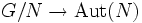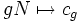# Quotient group acts on abelian normal subgroup

## Statement

Suppose$G$ is a group and$N$ is an Abelian normal subgroup. Then, the quotient group$G/N$ has a naturally induced action on$N$, i.e., there is a homomorphism:$G/N \to \operatorname{Aut}(N)$

given as follows:$gN \mapsto c_g$

where$c_g$ is conjugation by$g$ in$G$.

The action is faithful if and only if$N$ is also a self-centralizing subgroup.

We need$N$ to be normal for the conjugation action to define an automorphism of$N$, and we need$N$ to be Abelian for the map to be well-defined and independent of the choice of the coset representative.